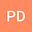An isotope ratio mass spectrometer (IRMS) for clumped isotope studies of carbon dioxide
•# Abstract

Rationale: Clumped isotope geochemistry is concerned with measuring the natural abundance of isotopic molecules, unit cells and other moieties that contain two or more rare stable isotopes. Accurate measurement of the abundance of such multiply substituted species using isotope ratio mass spectrometry (IRMS) techniques imposes stringent requirements on instrument performance in terms of overall sensitivity, linearity etc. that are difficult to achieve. To meet these requirements we have designed, constructed and evaluated the performance of a new IRMS that is optimised for clumped isotope ratio measurements of CO$$_{2}$$.

Methods: Following an analysis of the factors that limit the performance of existing IRMS instrument designs for clumped isotope measurements we determined an optimum instrument/magnet geometry using ion optic transfer matrix methods, and considering aberrations up to second order. Before the final engineering design the individual component ion optics (source and faraday collectors) were modelled using SIMION. The instrument has a 120$$^{o}$$, symmetric extended geometry with a gas dual-inlet. Construction is based on standard UHV principles. Instrument control and data acquisition is based on National Instruments compactRIO hardware and LabVIEW software.

Results: We demonstrate that the MIRA mass spectrometer has excellent sensitivity (<500 CO$$_{2}$$ molecules.ion$$^{-1}$$) combined with high abundance sensitivity and a linear response in terms of measured $$\Delta_{47}$$ over a 100‰ range in measured absolute isotope composition ($$\delta^{47}$$) with respect to the working reference gas of the mass spectrometer. Precision of measured $$\Delta_{47}$$ is at the shot noise limit of <0.01‰ for a standard measurement cycle of 80 minutes duration. Long term stability of the instrument and measurements is excellent.

Conclusions: The MIRA instrument can measure CO$$_{2}$$ isotopologues to a high precision (0.01‰ for $$\Delta_{47}$$). The instrument is linear and has sufficient sensitivity for both high sample throughputs (12 measurements per 24 hour cycle) and the analysis of gas samples down to 10$$^{-5}$$ moles in size.